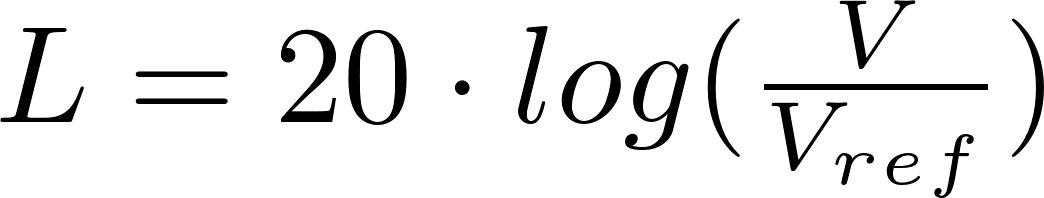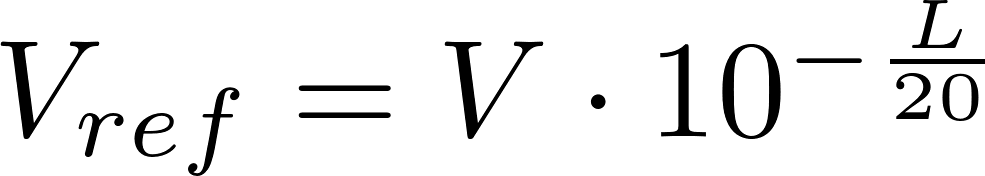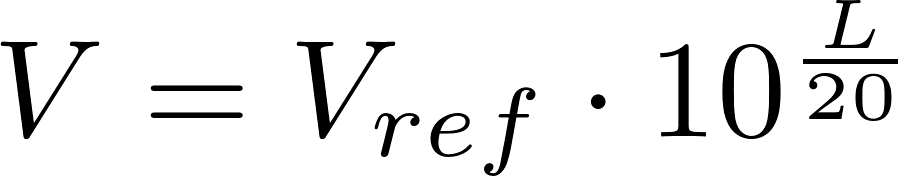# Decibel (voltage)

The decibel (dB) is used to express the ratio of two values on a logarithmic scale.

### Formulas

The following formula can be used to calculate the decibel value for two voltages or one of the voltages.L is the symbol for the decibel value and is measured in dB.
V is the symbol for voltage and is measured in volt (V).
V ref is the symbol for reference voltage and is measured in volt (V).

### Calculator

Enter two values to calculate the third.